• python中matplotlib画图导入到word如何保证分辨率
千次阅读
2018-11-19 12:38:28
更多相关内容
• matplotlib简单的画图练习 线形图 import matplotlib.pyplot as plt import numpy as np x = np.linspace(0, 2 * np.pi, 100) # 随机生成一个 0至100之间的数 间隔是 2*pi y1, y2 = np.sin(x), np.cos(x) # 给用 ...

matplotlib的安装
pip install matplotlib

matplotlib简单的画图练习

# 线形图

import matplotlib.pyplot as plt
import numpy as np

x = np.linspace(0, 2 * np.pi, 100) # 随机生成一个 0至100之间的数 间隔是 2*pi
y1, y2 = np.sin(x), np.cos(x)      # 给用 y1 y2 赋值
plt.title('sin & cos')             # 线形图标题 可选  可不写
plt.xlabel('x')                    # 建立数轴x 可选  可不写
plt.ylabel('y')                    # 建立数轴y 可选  可不写
plt.plot(x, y1)                    # 分别画出 x,y1所在的数值
plt.plot(x, y2)                    # 分别画出 x,y2所在的数值
plt.show()


线形 效果图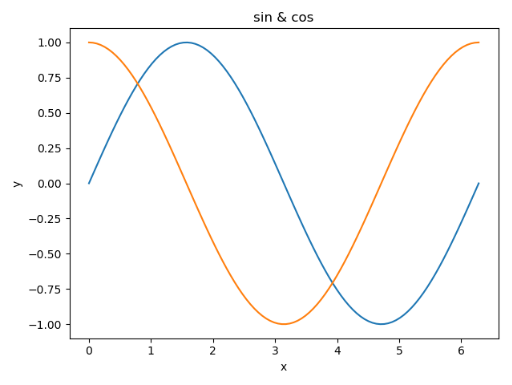# 条形图/柱状图

import matplotlib.pyplot as plt

name_list = ['A', 'B', 'C', 'D']
num_list = [1.5, 0.6, 7.8, 6]
# 条形图个数 参数 条形图颜色红绿蓝循环 每个条形的名称
plt.bar(range(len(name_list)), num_list, color='rgb', tick_label=name_list)
plt.show()


条形 效果图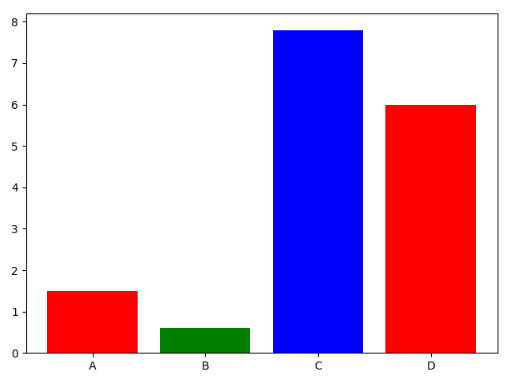# 堆叠柱状图 鸡肋

import matplotlib.pyplot as plt

name_list = ['A', 'B', 'C', 'D'] # 数据a
num_list = [1.5, 0.6, 7.8, 6] # 数据a
name_list1 = ['A', 'B', 'C', 'D'] # 数据b
num_list1 = [0.5, 1.6, 4.8, 3] # 数据b
# 条形图个数 参数 表名 可选 颜色黄色
plt.bar(range(len(name_list)), num_list, label='boy', fc='y')
# 条形图个数 参数 表名 可选 颜色黄色
plt.bar(range(len(name_list1)), num_list1, label='girl', fc='r')
plt.legend() # 堆叠
plt.show()


堆叠柱状 效果图 出现了覆盖的情况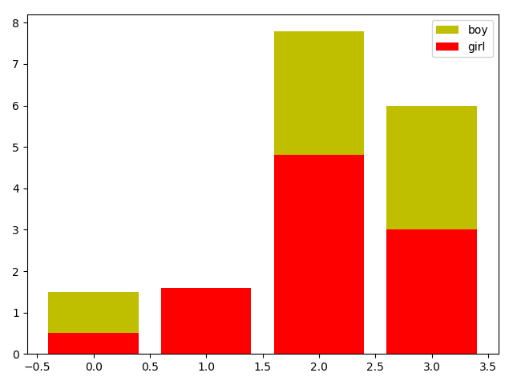# 并列柱状图 解决上面的堆叠柱状图的鸡肋情况

import matplotlib.pyplot as plt

name_list = ['A', 'B', 'C', 'D'] # 数据a
num_list = [1.5, 0.6, 7.8, 6] # 数据a
name_list1 = ['A', 'B', 'C', 'D'] # 数据b
num_list1 = [0.5, 1.6, 4.8, 3] # 数据b

x = list(range(len(name_list))) # 将name_list做成列表长度 x
totle_width, n = 0.4, 2 # 每个柱状图的宽度,一共两根柱状图
width = totle_width / n # 柱状图的间距总宽除以二
# x传入有几个柱状图 num_list传入柱状图的数据 width柱状图的宽度 label表名 fc颜色
plt.bar(x, num_list, width=width, label='boy', fc='b')
# 将每个x的值加上柱状图的宽度
for i in range(len(x)):
x[i] = x[i] + width
# 再次传入x的值个柱状图 num_list1传入柱状图数据 width柱状图宽度 label表名 fc颜色
plt.bar(x, num_list1, width=width, label='girl', fc='r')
plt.legend() # 重叠
plt.show()


并列柱状 效果图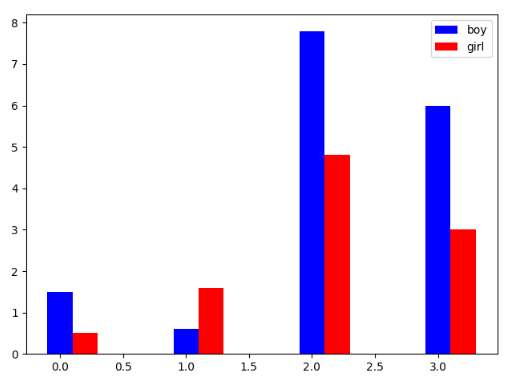# 饼状图

import matplotlib.pyplot as plt

labels = 'A', 'B', 'C', 'D', 'E' # 传入饼状图的表名
faces = [15, 30, 55, 44.44, 10] # 传入饼状图的值
plt.axes(aspect=1) # 画一个单位圆
explode = [0, 0, 0, 0.2, 0] # 选择需要取出的 那部分
# 　　　传入数据个数　　 传入表名 以浮点数显示 阴影开 起始角 选择需要取出那块
plt.pie(x=faces, labels=labels, autopct='%3.1f %%', shadow=True, startangle=90, explode=explode)
plt.show()


饼状 效果图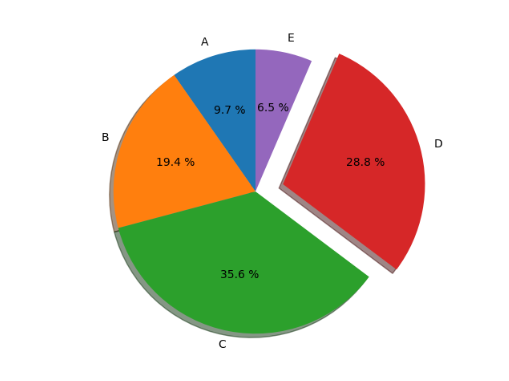展开全文• matplotlibpython中最常用的绘图第三方库，基本可以实现绝大多数图形的绘制（很优秀），本文将分享一种高效的matplotlib绘图流程，让你轻轻松松五步作图。 2. 常规模式 import matplotlib.pyplot
• 今天小编就为大家分享一篇Python matplotlib 画图窗口显示到gui或者控制台的实例，具有很好的参考价值，希望对大家有所帮助。一起跟随小编过来看看吧
• python matplotlib画图使用colorbar工具自定义颜色 colorbar（draw colorbar without any mapple/plot） 自定义colorbar可以画出任何自己想要的colorbar，自由自在、不受约束，不依赖于任何已有的图(plot/mappable)...
• python中使用matplotlib画图，里面的中文会显示乱码方块。 解决方法 这是由于matplotlib默认使用的字体不包含中文字符引起的，可以通过将中文字符加入到默认字体解决。 前提：查找本地都有哪些中文字体 打开...

# 问题

在python中使用matplotlib画图，里面的中文会显示乱码方块。

# 解决方法

这是由于matplotlib默认使用的字体中不包含中文字符引起的，可以通过将中文字符加入到默认字体中解决。

#### 前提：查找本地都有哪些中文字体

打开matplotlib字体，默认为C:\Users\用户名\.matplotlib，打开fontList.json，查找中文字体如simHei,song,kai等，分别对应简体黑、宋体、楷体等。

查找到之后，其对应的name字段值即为我们接下来将要设置的中文字体名，复制下来，比如中宋字体STZhongsong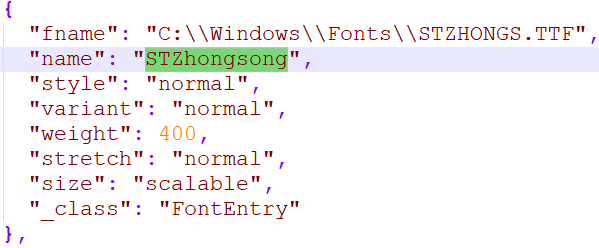### 方法一：代码解决

在脚本开头加入以下语句即可：

from pylab import mpl
mpl.rcParams['font.sans-serif'] = ['STZhongsong']    # 指定默认字体：解决plot不能显示中文问题
mpl.rcParams['axes.unicode_minus'] = False           # 解决保存图像是负号'-'显示为方块的问题


### 方法二：永久解决

运行下面的代码：

import matplotlib
print(matplotlib.matplotlib_fname())


会输出配置文件路径，如：

C:\Users\gong\AppData\Local\Continuum\anaconda3\lib\site-packages\matplotlib\mpl-data\matplotlibrc


打开此文件，找到#font.family:#font.sans-serif:开头的这两行，将两行的注释#去掉，并在font.sans-serif:后添加自己想加入的中文字体名，如：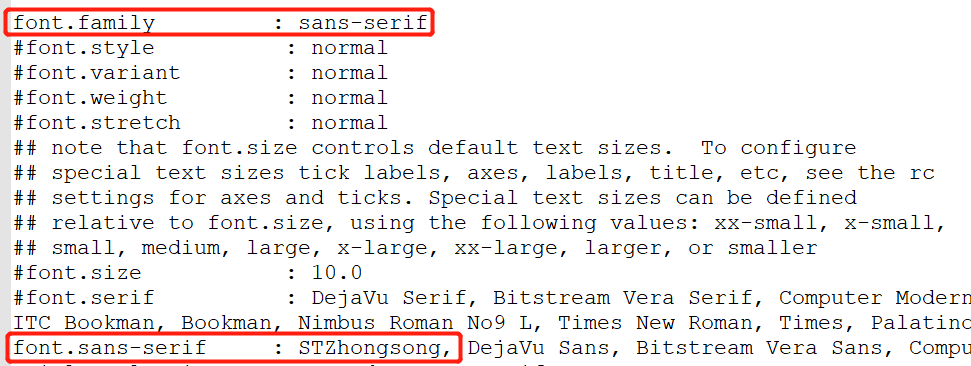然后保存文件，重新运行python环境即可，不必在代码中做出任何修改。

展开全文• 在 Ubuntu 18.0 系统上使用 matplotlib 画图，其中在添加中文作为标题或者坐标轴名称时，显示为乱码，如下所示： 这是因为在 Ubuntu 系统上默认没有中文字体。所以需要手动下载一个字体，并且添加到 matplotlib 库...

在 Ubuntu 18.0 系统上使用 matplotlib 画图，其中在添加中文作为标题或者坐标轴名称时，显示为乱码，如下所示：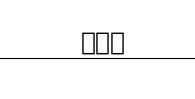这是因为在 Ubuntu 系统上默认没有中文字体。所以需要手动下载一个字体，并且添加到 matplotlib 库的字体路径下。

##### 1、下载字体

比如我在网上下载的是 黑体：##### 2、找到 matplotlib 字体所在的位置

进入 Python 的交互界面，输入如下命令：

import matplotlib
matplotlib.matplotlib_fname()


会输出一个路径，那就是 matplotlib 字体库的大致位置，为什么是大致位置呢，因为只是一部分。
当然有的环境因为是使用的虚拟环境，不要紧，因为后面一段路径都是一样的。
以下是这个目录的后面一截：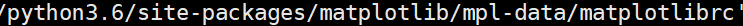然后退出交互环境，根据这个完整路径去到这个目录的前一级，也就是：

cd ..../python3.6/site-packages/matplotlib/mpl-data/
#前面省略了一截，真实输入的时候补全


进入到这个目录，然后输入 ls -l 显示当前的目录：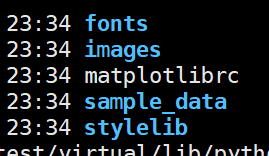然后，进入这个目录下的 **/fonts/ttf/**路径。

cd fonts/ttf


然后再 ls -l ，可以发现都是后缀为 .ttf 的字体文件。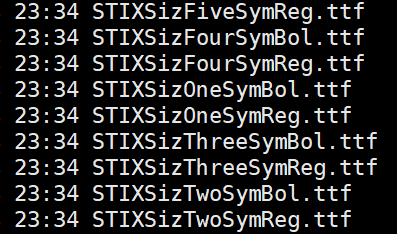##### 3、将下载的字体上传到上述文件夹

将字体上传到该文件夹以后，清除 matplotlib 的缓存信息，

rm ~/.cache/matplotlib/ -rf


然后在画图前，引入该字体，再画图，就可以看到中文不是乱码了，以下是测试代码：

import matplotlib
import matplotlib.pyplot as plt
import random
#引入我们刚刚上传的字体， SimHei 为字体名称
matplotlib.rcParams['font.family'] = "SimHei"
x = list(range(7))
y = random.sample(range(1,10), 7)
plt.scatter(x, y)
plt.title('散点图', fontsize=18)
plt.savefig('/home/hunter/scatter.png')


如下是示意图：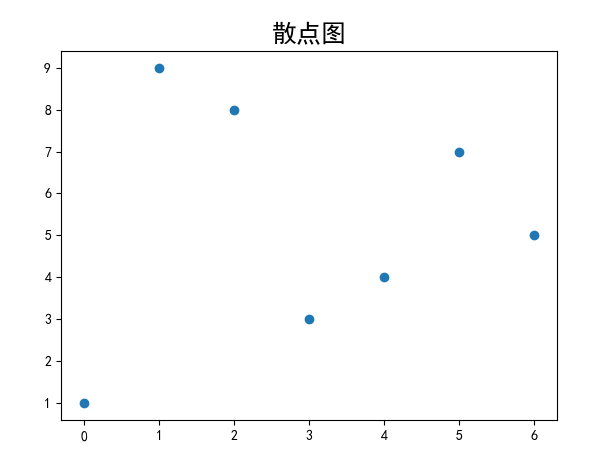至此，关于 Ubuntu 上中文字体的乱码问题就解决啦！！！

展开全文• import matplotlib.pyplot as plt ax = [] # 定义一个 x 轴的空列表用来接收动态的数据 ay = [] # 定义一个 y 轴的空列表用来接收动态的数据 plt.ion() # 开启一个画图的窗口 for i in range(100): # 遍历0-99的值 ...
• Matplotlib.pyplot是用来画图的方法，类似于matlabplot命令，用法基本相同。本文档主要讲述的是python matplotlib画图
• 下面小编就为大家带来一篇基于Linux系统中python matplotlib画图的中文显示问题的解决方法。小编觉得挺不错的，现在就分享给大家，也给大家做个参考。一起跟随小编过来看看吧
• 本文讲的是与plot(), scatter(), pyplot()等绘图函数常用的参数。 目录1 marker 标号1.1 相关参数2 linestyle 线的样式3 linewidth 线宽4 label 图例5 alpha 透明度6 color 颜色创建自己的colormapmatplotlib....
• 主要介绍了python matplotlib画图实例代码分享，具有一定借鉴价值,需要的朋友可以参考下
• 莫烦PYTHON——Matplotlib画图教程 学习心得（2）3 画图种类3.1 Scatter 散点图3.2 Bar 柱状图3.3 Contours 等高线图3.4 Image 图片3.5 3D 数据4 多图合并显示4.1 Subplot 多合一显示4.2 Subplot 分格显示4.3 图中图...
• 主要介绍了Python使用matplotlib绘图无法显示中文问题的解决方法,结合具体实例形式分析了Python使用matplotlib绘图时出现中文乱码的原因与相关解决方法,需要的朋友可以参考下
• 主要介绍了解决Linux系统中python matplotlib画图的中文显示问题,需要的朋友可以参考下
• 附件整理了python中数据展示使用到的库、函数、方法等的参数和用法，属于笔记类。知识点较散，用文字不太好描述，所以使用了思维导图的形式。希望对大家有帮助。
• 本文实例讲述了Python matplotlib画图与中文设置操作。分享给大家供大家参考，具体如下： 采用matplotlib作图时默认设置下是无法显示中文的，例如编写如下python脚本， #-*- coding: utf-8 -*- from pylab import *...
• pythonmatplotlib画图时，往往需要加图例说明。如果不设置任何参数，默认是加到图像的内侧的最佳位置。 import matplotlib.pyplot as plt import numpy as np x = np.arange(10) fig = plt.figure() ax = plt....
• Python中matplotlib画图正常显示设置 import matplotlib.pyplot as plt %matplotlib inline plt.rcParams['font.sans-serif']=['SimHei'] #用来正常显示中文标签 plt.rcParams['axes.unicode_minus']=False #用来...
• python中会使用很多图标的东西作为可视化输出的必不可少的内容。matplotlib提供了很好的图形化显示。 下面就如何快速安装matplotlib做一下简要的说明： 博主用的python版本是3.8.2 win + R 输入 python 一般情况...
• MatplotlibPython提供的一个二维绘图库，所有类型的平面图，包括直方图、散点图、折线图、点图、热图以及其他各种类型，都能由Python制作出来。本文主要介绍了关于Python利用matplotlib.pyplot绘图时如何设置坐标...
• 1、使用%pylab查看matplotlib后端 2、查看自己的配置支持什么 3、设置使用自己的配置所支持的 import matplotlib matplotlib.use('TkAgg')
• 如果你想要在Linxu获得一个高效、自动化、...这个教程将提供几个用matplotlib画图的例子。 特性 支持众多的图表类型，如：bar,box,contour,histogram,scatter,line plots…. 基于python的语法 集成Numpy科学计算包
• import xlrd import openpyxl from tkinter import filedialog #路径选择 from tkinter import * import matplotlib import matplotlib.pyplot as plt from matplotlib.backends....from matplotlib.figure import Fig
• 主要介绍了使用python matplotlib 画图导入到word如何保证分辨率的例子，具有很好的参考价值，希望对大家有所帮助。一起跟随小编过来看看吧...